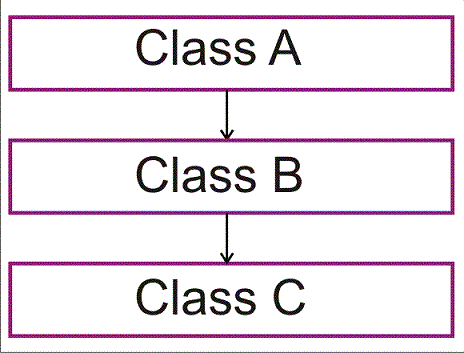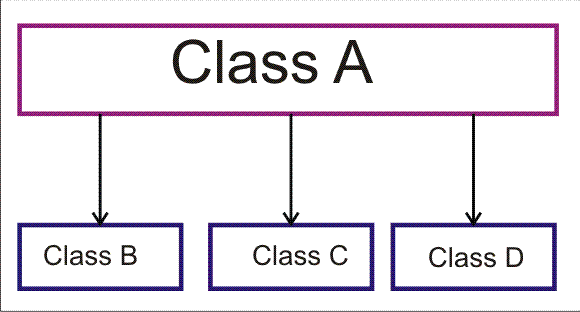# C++ language

Types of Inheritance (Hierarchal, Multilevel, Hybrid)
 Previous Home Next

Hierarchal Inheritance

In this inheritance ,is use hierarchal design. Here a base class is derived to other two or more derived class and then derived class is become base class for next level derived class. This include multilevel inheritance .### Structure of Hierarchal Inheritance:

``` # include<iostream.h>
class base
{
....
.....                         // class body
.....
};
class derived1:public base
{
....
.....                       // class body
.....
};
class derived2:public base
{
....
.....                       // class body
.....
};
class derived3:public base
{
....
.....                        // class body
.....
};
class derived2_a:public derived2
{
....
.....                        // class body
.....
};
class derived2_b:public derived2
{
....
.....                       // class body
.....
};
class derived2_c:public derived2
{
....
.....                       // class body
.....
};
```

### Structure of Hierarchal Inheritance:

```#include<iostream>
const int num = 20 ;
class student                          // Base class
{
private: char F_name[num] , L_name[num] ;
int age, int roll_no ;
public:
void Enter_data(void)
{
cout << "\n\t Enter the first name: " ; cin >> F_name ;
cout << "\t Enter the second name: "; cin >> L_name ;
cout << "\t Enter the age: " ; cin >> age ;
cout << "\t Enter the roll_no: " ; cin >> roll_no ;
}
void Display_data(void)
{
cout << "\n First Name = " << F_name ;
cout << "\n Last Name = " << L_name ;
cout << "\n Age = " << age ;
cout << "\n Roll Number = " << roll_no ;
}
};
class arts : public student           //derived class of same base class
{
private:
char subject_name1[num] ;
char subject_name2[num] ;
char subject_name3[num] ;
public:
void Enter_data(void)
{
cout << "\t Enter the subject1 : "; cin >> subject_name1 ;
cout << "\t Enter the subject2  "; cin >> subject_name2 ;
cout << "\t Enter the subject3  "; cin >> subject_name3 ;
}
void Display_data(void)
{
cout << "\n Subject1  = " << subject_name1 ;
cout << "\n Subject2 = " << subject_name2 ;
cout << "\n Subject3  = " << subject_name3 ;
}
};
class arts : public student           //derived class of same base class
{
private:
char s_subject_name1[num] ;
char s_subject_name2[num] ;
char s_subject_name3[num] ;
public:
void Enter_data(void)
{
cout << "\t Enter the subject1 : "; cin >> s_subject_name1 ;
cout << "\t Enter the subject2  "; cin >> s_subject_name2 ;
cout << "\t Enter the subject3  "; cin >> s_subject_name3 ;
}
void Display_data(void)
{
cout << "\n Subject1  = " << s_subject_name1 ;
cout << "\n Subject2 = " << s_subject_name2 ;
cout << "\n Subject3  = " << s_subject_name3 ;
}
};
class commerce : public student         //derived class of same base class
{
private: char c_subject_name[num],c_subject_name2[num],c_subject_name3[num] ;
public:
void Enter_data(void)
{
cout << "\t Enter the subject1: ";
cin >> c_subject_name;
cout << "\t Enter the subject2 : ";
cin >>c_subject_name2 ;
cout << "\t Enter the subject3 : ";
cin >>c_subject_name3 ;
}
void Display_data(void)
{
cout << "\n Subject1  = " << c_subject_name ;
cout << "\n Subject2  = " <<c_subject_name2 ;
cout << "\n Subject3  = " <<c_subject_name3 ;
}
};

void main(void)
{
arts a ;
cout << "\n  details of the arts student\n" ;
a.Enter_data( );
cout << "\n  details of the arts student\n" ;
a.Display_data( );
science s ;
cout << "\n  details of the science student\n" ;
s.Enter_data( );
cout << "\n  details of the science student\n" ;
s.Display_data( );
commerce c ;
cout << "\n details of the commerce student\n" ;
c.Enter_data( );
cout << "\n details of the commerce student\n";
c.Display_data( );
}
```

### Multilevel Inheritance

In multilevel inheritance where a derived class inherit the property on one base class and that derived class is become the base class for another derived class.

For example A is a base class and B inherit the property of A here B is a derive class and another class C is inherit the property of class B now class B become the base class for class C and class C is derived class For class B. class A indirectly base Class of Class C and class C indirect derived class for class.### Structure of Hierarchal Inheritance:

```# include<iostream.h>
class base
{
....
.....                      // class body
.....
};
class derived1:public base        // derived from base class
{
....
.....                      // class body
.....
};
class derived2:public derived1    // derived from derived class
{
....
.....                      // class body
.....
};
```

### Structure of Multilevel Inheritance:

```# include<iostream.h>
class student
{
protected:
int roll_num;
public:
void enter_number(int a)
{
roll_num=a;
}
void display_number(void)
{
cout<<
"Enter roll number is"<<roll_num<<
"\n";
}
};
class test : public student //First level derivation
{
protected:
float subject1:
float subject2;
public:
void enter_marks(float x, float y)
{
subject1=x;
subject2=y;
}
void display_marks(void)
{
cout<<"marks of subject1"<<subject1<<"\n";
cout<<"marks of subject2"<<subject2<<"\n";
}

};
class result : public test												         //Second level derivation
{
float total;
public:
void display(void)
{
total=subject1+subject2;
display_number();
display_marks();
cout<<"total ="<<total<<"\n";
}
};
int main()
{
result re;
re.enter_number(100);
re.enter_marks(76.5,77.5);
re.display();
return 0;
}
};
```

### Hybrid Inheritance:

In hybrid Inheritance , this is combination of two or more  type of inheritance . but in hybrid inheritance the error will occur is known as ambiguity . Where same function name can have more then one class then compiler confused to identify called function.Structure of Hybrid Inheritance:
```# include<iostream.h>
class student
{
protected:
int roll_num;
public:
void enter_number(int a)
{
roll_num=a;
}
void display_number(void)
{
cout<<"Enter roll number is"<<roll_num<<"\n";
}
};
class test : public student//First level derivation
{
protected:
float subject1:
float subject2;
public:
void enter_marks(float
x, float y)
{
subject1=x;
subject2=y;
}
void display_marks(void)
{
cout<<
"marks of subject1"<<subject1<<
"\n";
cout<<
"marks of subject2"<<subject2<<
"\n";
}
};
class sport
{
protected:
float score;
public:
void enter_ score(int i)
{
score=i;
}
void display_ score(void)
{
cout<<"Enter  score is"<< score<<"\n";
}
class result : public test
{
float total;
public:
void display(void)
{
total=subject1+subject2+ score;
display_number();
display_marks();
display_ score();
cout<<"total ="<<total<<"\n";

};
int main()
{
result re;
re.enter_number(1234);
re.enter_marks(27.5,33.0);
re.enter_score(6.0);
re.display();
return 0;
}
};
```

Oututp

```Enter roll number is 1234
marks of subject1 27.5
marks of subject2 33
Enter score is 6
total=66.5
```